# AreaPage 1

#### WATCH ALL SLIDES

Slide 1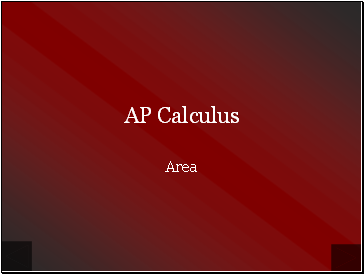AP Calculus

Area

Slide 2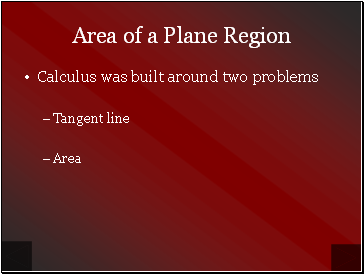## Area of a Plane Region

Calculus was built around two problems

Tangent line

Area

Slide 3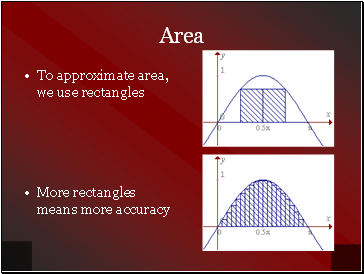## Area

To approximate area, we use rectangles

More rectangles means more accuracy

Slide 4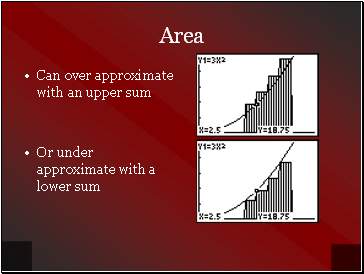Area

Can over approximate with an upper sum

Or under approximate with a lower sum

Slide 5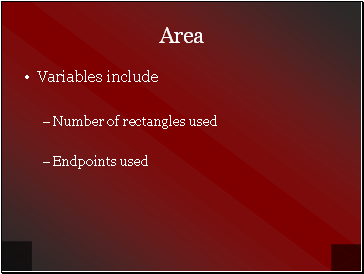Area

Variables include

Number of rectangles used

Endpoints used

Slide 6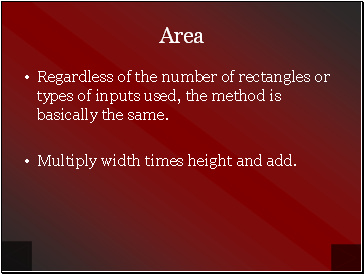Area

Regardless of the number of rectangles or types of inputs used, the method is basically the same.

Multiply width times height and add.

Slide 7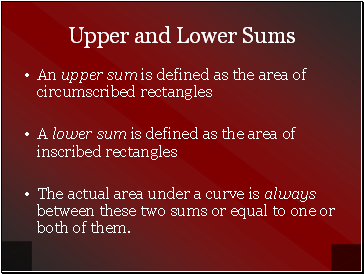## Upper and Lower Sums

An upper sum is defined as the area of circumscribed rectangles

A lower sum is defined as the area of inscribed rectangles

The actual area under a curve is always between these two sums or equal to one or both of them.

Slide 8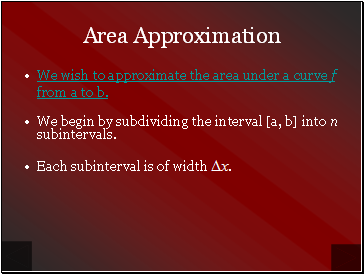## Area Approximation

We wish to approximate the area under a curve f from a to b.

We begin by subdividing the interval [a, b] into n subintervals.

Each subinterval is of width .

Slide 9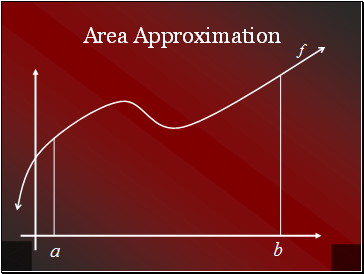Area Approximation

Slide 10Area Approximation

We wish to approximate the area under a curve f from a to b.

We begin by subdividing the interval [a, b] into n

subintervals of width .

Minimum value of f in the ith subinterval

Maximum value of f in the ith subinterval

Slide 11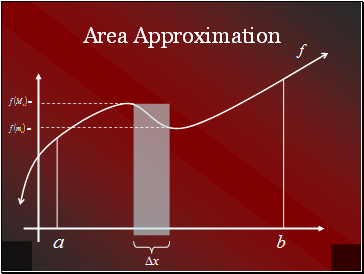Area Approximation

Slide 12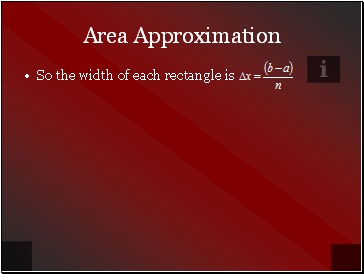Area Approximation

So the width of each rectangle is

Slide 13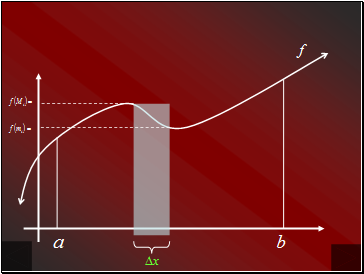Slide 14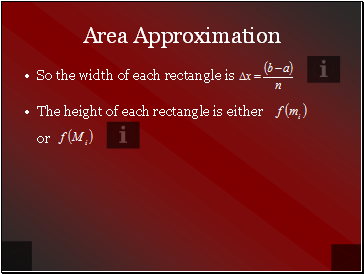So the width of each rectangle is

The height of each rectangle is either

or

Area Approximation

Slide 15Go to page:
1  2